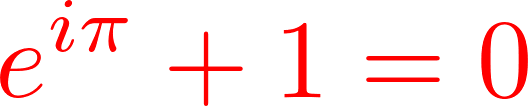# Creating a PNG image of a formula in generativepy

By Martin McBride, 2022-08-30
Tags: generativepy tutorial formula
Categories: generativepy generativepy tutorialIn this article we will look at the simplest way to render a Latex formula as a PNG image using generativepy.

We will use the rasterise_formula function of the formula module. First, we will look at how a formula is defined.

## Latex

Latex provides a way to define mathematical formulas in plain text. For example, this Latex formula:

x=\frac{-b\pm\sqrt{b^2-4ac}}{2a}


Defines the well-known formula for the solutions to a quadratic equation. When this definition is rendered, it looks something like this:We won't go into details about how Latex works here, there are many examples online. This article just looks at how to render Latex as an image.

## generativepy

generativepy is a Python library for creating generative art and mathematical illustrations in code. It has many features, but the one we will look at here is how to render Latex as a PNG image.

See the generativepy reference for details on how to install the library. You will also need NumPy installed, and the command line programs latex and dvipng.

## Converting Latex to PNG

Here is the Python code to convert a couple of equations to images:

from generativepy.color import Color
from generativepy.formulas import rasterise_formula

rasterise_formula("create-png-1", r"e^{i \pi}+1 = 0", Color("red"), dpi=800)
rasterise_formula("create-png-2", r"\frac{\cancel{a}b}{\cancel{a}c} = 0",
Color("green"), packages=["cancel"])


After importing a couple of required modules from generativepy, we just need to call rasterise_formula, passing in the three required arguments:

• name - the base name that will be used to create the PNG file. This file will always be created in the working folder.
• formula - the Latex formula string. This is just the formula definition, it should not be enclosed in \$ symbols, and you do not need begin or end text.
• The colour of the text, as a Color object (defined in generativepy.color). You can use any CSS named colours, and there are also lots of other ways to define a colour.

The first call above adds an optional dpi parameter that controls the size of the image. The actual size of the image is determined by the formula itself, the dpi parameter acts as a scale factor. The default is 600, but you can make the image bigger or smaller by using a larger or smaller dpi value. Here is the result:Latex contains many optional packages that include extra features. The second call to rasterise_formula shows how we can use packages when rendering Latex. We add a packages argument consisting of a list of package names (in this case just on, the cancel package). We can then use the Latex \cancel operator in our function, which draws diagonal lines through the a characters to cancel them out. Here is the result:## Summary

It is very easy to render Latex formulas in Python with the generativepy library. In a future article, we will see how to add formulas to graphs and videos.

If you found this article useful, you might be interested in the book NumPy Recipes or other books by the same author.